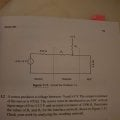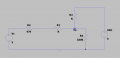help, right equation to calculate a resistive circuit?

TarikElec

Joined Oct 17, 2019
61
I have reading started reading the book : Ten Essential Skills for Electrical Engineers by BARRY L.DORR. in the first chapter : How to design resistive circuit. int the proposed exercise 1.2 where I am stuck.
I have assumed that the Voffset=VA is 5*3.3/8=2.0625V for Vs=0, to make my calculation easy.
I have also calculated the gain of G=3.3/8=2.0625 which is exactly as the Voffset.
after a couple of calculation trial using the thevening theorem for the AC mode. I ended up both in the wrong value of R1 and R2, so it is for the simulation and compared to the result provided by the book R1=930 and R2=1400.
my result for R1=R3*(1-G-G^2)/G^2.=8000 roughly which is wrong.
below you can see the exercise and how I named it.

I am still wondering where I did make a mistake in the begining because I have split the solution into two:
1-AC mode:Rth=R2//R3, Vth=Vs*Rth/[Rth+R1+R4] with Vth/Vs=3.3/8. I took R1=fc(R2,G,R2,R3,R4) then replaced it in the DC mode
2-DC mode :Va=Vdc*[R3//(R1+R4)]/[3//(R1+R4)/+R2], with Va/Vdc=2.0625/5=3.3/8Last edited by a moderator:

Alec_t

Joined Sep 17, 2013
12,485
I don't know the context of the exercise, but why are you doing an AC analysis?. No AC parameters are given for the sensor.

•TarikElec

TarikElec

Joined Oct 17, 2019
61
I don't know the context of the exercise, but why are you doing an AC analysis?. No AC parameters are given for the sensor.
This is a coupling circuit design.in the AC mode: I have the Vs which is a voltage varying from -5v to 3v, which makes me make a gain of G=3.3/8. also calculatin gthe Vth and Rth(thevenin) in AC mode I could extract R1 in function of the rest know value and R2.
I can make a pic of his examples if you want

Last edited:

MisterBill2

Joined Jan 23, 2018
10,602
There are two conditions to meet: At Vs=-5, Va=0.0, and at Vs=+3, Va=3.3 volts.
Given that there are no reactive components, the resistor values should be the same for an AC variation of Vs. Thevenin is one approach, but since all of the end voltages are known it can also be done by super-position.
The voltage at Va is known when Vs = -5 (Va=0) and when Vs =+3, (Va=+3.3) Solving for the current in R2 when Va=0, and then adding the current in R2 with Vs=-5 will give the total current. And the current in R1 can then be calculated.

TarikElec

Joined Oct 17, 2019
61
Sir, I have tried the superposition and I could not get results. the thevenin theorem is used because in AC, we can add a capacitor and if we add capacitor the other resistor of the source is neglected in DC, wich facilitate the calculation.
my concern did I make wrong assumptions in the mentioned method?

TarikElec

Joined Oct 17, 2019
61
I have found that the solution is using the thevenin theorem and I made a mistake in the calculation and the resutl are exactl the same as presented in the book. R1=R3*(1-2*G)/G^2

MisterBill2

Joined Jan 23, 2018
10,602
OK, the reference to "gain" was rather confusing,because there are no active devices in the circuit. Using the term "gain" will probably confuse others as well.
And if I were grading a test I would as to see the steps involved in obtaining that expression for the value of R1. "G" is a fairly complex term in this case and I have not seen it used in DC circuit analysis of circuits without active components.

•TarikElec

TarikElec

Joined Oct 17, 2019
61
OK, the reference to "gain" was rather confusing,because there are no active devices in the circuit. Using the term "gain" will probably confuse others as well.
And if I were grading a test I would as to see the steps involved in obtaining that expression for the value of R1. "G" is a fairly complex term in this case and I have not seen it used in DC circuit analysis of circuits without active components.
I would soon make a screen shot of the book in that expression.
to summerise, I have two equations. on ein AC mode where I am supposed to have a gain between the two IC an dthe other equation is to have DC offset. i took R1 from AC gain and wrote it in function of R2. then replace it in DC mode and I got R2 value, from there , I got R1.

MisterBill2

Joined Jan 23, 2018
10,602
OK, but please understand that superposition does work for the circuit, and it is convenient because the voltages are all known. And it is important to add all of the resulting currents correctly.
Now with "two ICs", I am guessing that they will be op-amps, possibly perfect ones, with well defined input and output resistances. It will be interesting to see that problem, and if there is no variation of any of the voltages then the AC analysis should match the DC analysis.